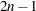TYPE=TREE Data Sets

Some clustering procedures produce TYPE=TREE data sets. For example, in PROC CLUSTER, a TYPE=TREE data set contains one observation for each observation in the input data set, plus one observation for each cluster of two or more observations (that is, one observation for each node of the cluster tree). The total number of output observations is usually, where n is the number of input observations. The density methods might produce fewer output observations when the number of clusters cannot be reduced to one.

In PROC VARCLUS, the OUTTREE= data set contains one observation for each variable clustered plus one observation for each cluster of two or more variables—that is, one observation for each node of the cluster tree. The total number of output observations is between n and, where n is the number of variables clustered. See Chapter 30, The CLUSTER Procedure, and Chapter 96, The VARCLUS Procedure, for details.